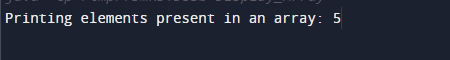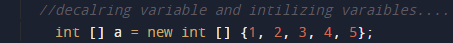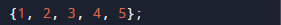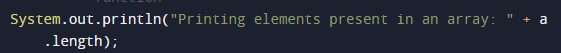# Java Program to print number of elements present in an array

In this tutorial you will learn about the Java Program to print number of elements present in an array and its application with practical example.

In this tutorial, we will learn to create a Java to Program Find 2nd Smallest Number in an array using java programming.

## Prerequisites

Before starting with this tutorial, we assume that you are best aware of the following Java programming concepts:

• Java Operators.
• Basic Input and Output function in Java.
• Class and Object in Java.
• Basic Java programming.
• If-else statements in Java.
• For loop in Java.

### Algorithm

• STEP 1: start
• 2: declare and initiate array = {1,2,3,4,5}.
• 3: Find length using length function ex=> a.length
• 4: exit.

## Java Program to print number of elements present in an array

In this program we will find number of element in an array using length function. We would first declared and initialized the required variables. Next, we will find length of an array element in an array. Lets have a look at the code.

## OutputIn the above program, we have first declared and initialized a set variables required in the program.

• a[]= it will hold array values.

After declaring variables we initiate values in  an array[].In this our program, we will count  the element and print number of elements present in an array.

As we can see that number of elements present in an array can be found by calculating the length of given array. We can clearly see that length of a function is 5 so here we will use length function to calculate length.So Length in above array is 5. Then number of elements present in the array are 5.Finally we will print the result as shown in image above..

In this tutorial we have learn about the Java Program to print number of elements present in an array and its application with practical example. I hope you will like this tutorial.